LINE CALCULATION

This is the main equation :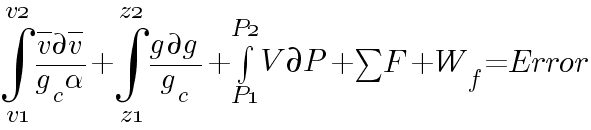Error must be less 1% of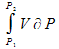Solve by iteration :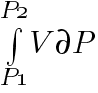This is the variation of volume by pressure , this expresion is converter into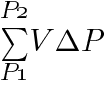where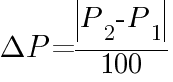if state is gas then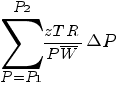if state is liquid then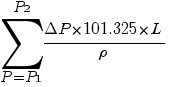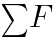This is the varation of friction factor by length of pipe.Friction factor is considerate a constant.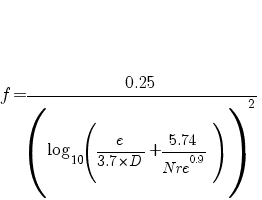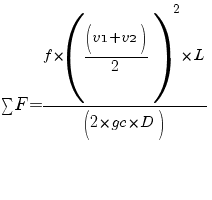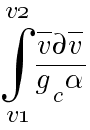This is the varation of velocity by velocity. gc and alpha is considerated constants.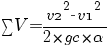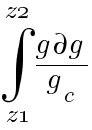This is the variation of gravity by grabity, this expresion is a constant. =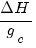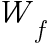This expresion is considerated zero.